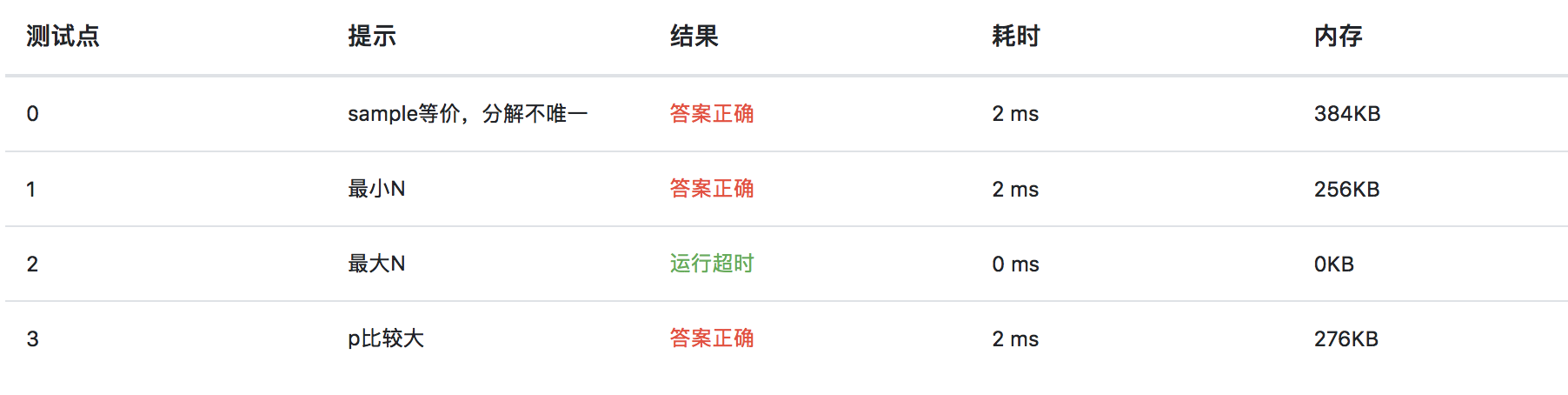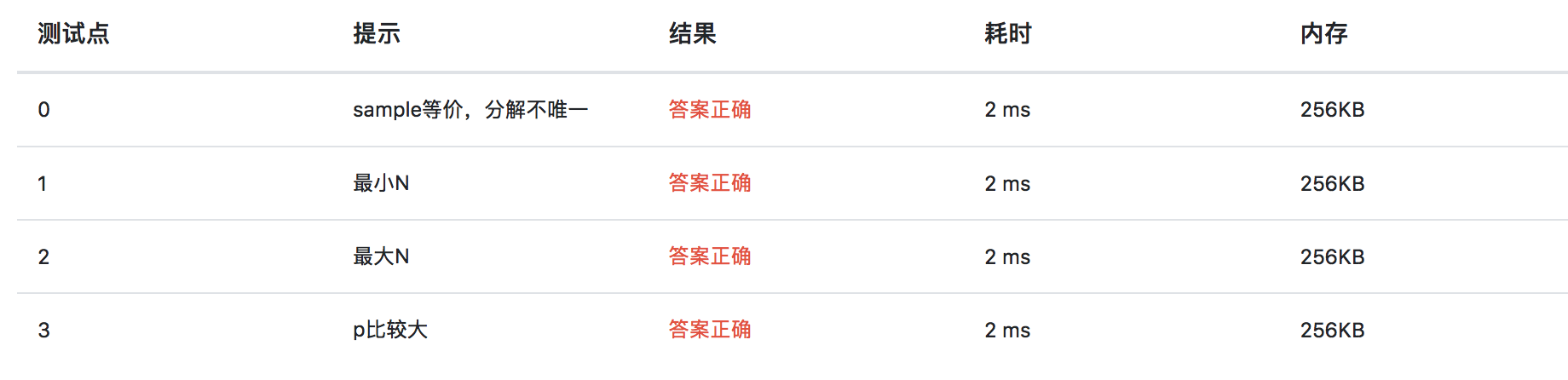# 实验4-2-3 验证“哥德巴赫猜想” （20 分）

24

#### 输出样例：

24 = 5 + 19

#include<stdio.h>

//检查一个数是否素数，是就输出1，否就输出0
int isprime(double n){
int isprime = 1;
if(n < 2){
isprime = 0;
}
for (int i = 2; i <= n/2; i++){
if((int)n%i == 0){
isprime = 0;
break;
}
}
return isprime;
}

int main(){
double n;
scanf("%lf", &n);
double p=2, q;
while(1){
q = n - p;
if(isprime(p)&&isprime(q)){
printf("%.0f = %.0f + %.0f\n", n, p, q);
break;
}
p++;
}
return 0;
}#include<stdio.h>
#include<math.h>

//检查一个数是否素数，是就输出1，否就输出0
int isprime(int n){
int isprime = 1;
if(n == 1 || (n%2 == 0 && n != 2)){
isprime = 0;
}
for (int i = 3; i <= sqrt(n); i += 2){
if(n%i == 0){
isprime = 0;
break;
}
}
return isprime;
}

int main(){
int n;
scanf("%d", &n);
int p, q;
for(p = 2; p<n; p++){
q = n - p;
if(isprime(p)&&isprime(q)){
printf("%d = %d + %d\n", n, p, q);
break;
}
}
return 0;
}